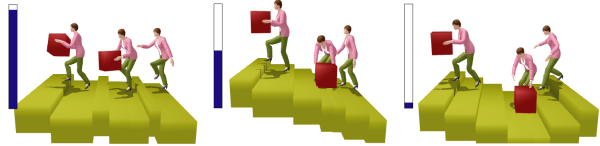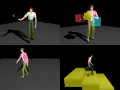# Statistical Interpolation of Human Motion Data

## Geostatistical Motion Interpolation### Abstract

A common motion interpolation technique for realistic human animation is to blend similar motion samples with weighting functions whose parameters are embedded in an abstract space. Existing methods, however, are insensitive to statistical properties, such as correlations between motions. In addition, they lack the capability to quantitatively evaluate the reliability of synthesized motions. This paper proposes a method that treats motion interpolations as statistical predictions of missing data in an arbitrarily definable parametric space. A practical technique of geostatistics, called universal kriging, is then introduced for statistically estimating the correlations between the dissimilarity of motions and the distance in the parametric space. Our method statistically optimizes interpolation kernels for given parameters at each frame, using a pose distance metric to efficiently analyze the correlation. Motions are accurately predicted for the spatial constraints represented in the parametric space, and they therefore have few undesirable artifacts, if any. This property alleviates the problem of spatial inconsistencies, such as foot-sliding, that are associated with many existing methods. Moreover, numerical estimates for the reliability of predictions enable motions to be adaptively sampled. Since the interpolation kernels are computed with a linear system in real-time, motions can be interactively edited using various spatial controls.

### Publications

1. Tomohiko Mukai and Shigeru Kuriyama, "Geostatistical Motion Interpolation", ACM Transactions on Graphics, Vol. 24, Issue. 3, pp.1062-1070, 2005.
paper (PDF: 4.2MB, preprint)
presentation slide (PowerPoint(zip): 3.3 MB, without movies)

### ResultsComplete SIGGRAPH 2005 video QuickTime: 51.4 MB with Audio 640×480, 5:00

### Sample code & motion data

1. Sample code of kriging (zip: 16 KB, C++, cross platform), Reference (doxygen)
NOTICE: You will need GNU Scientific Library to compile.
2. Sample motions

Please feel free to send me questions if you would like.